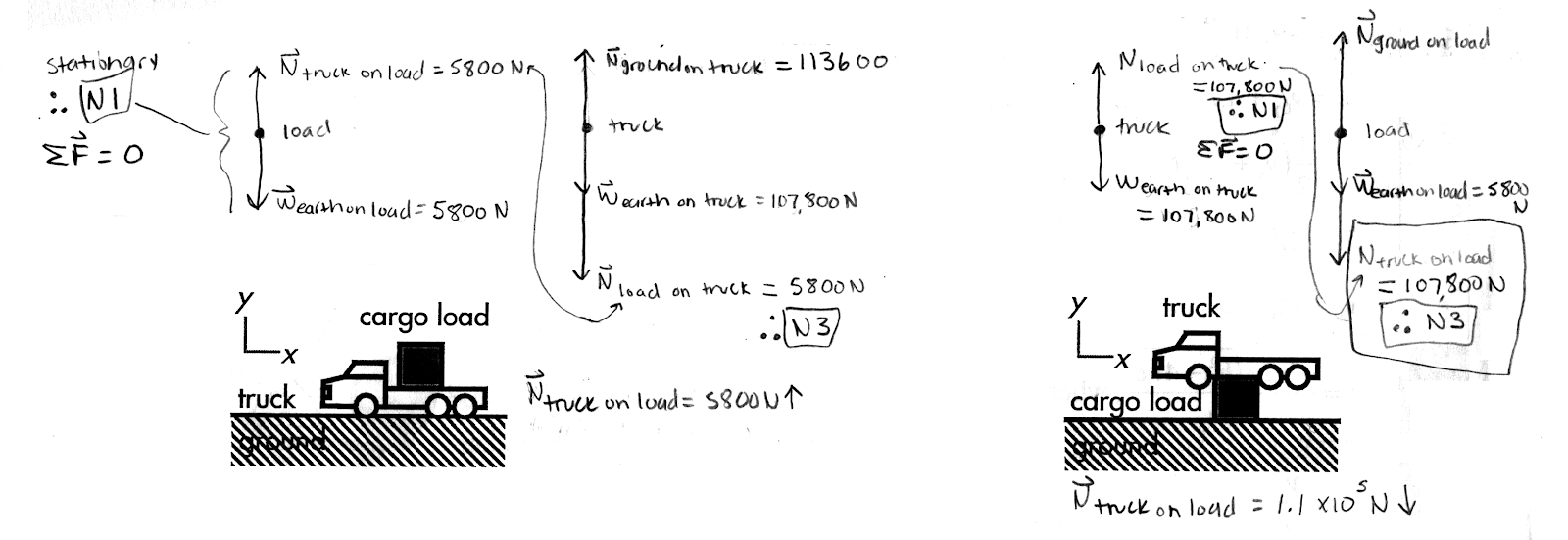## 20191011

Physics 205A Midterm 1, fall semester 2019
Cuesta College, San Luis Obispo, CA

A Physics 205A student draws a (correct) free body diagram for a 600 kg cargo load resting on a stationary 11,000 kg truck with these two forces[*]:
Weight force of Earth on cargo load (5,800 N, downwards),
Normal force of truck on cargo load (5,800 N, upwards).
This student additionally claims that "this [free body diagram for the cargo load] would change if the truck was on top of the cargo load." Discuss why both the magnitude and direction of the normal force of truck on the cargo load would change if the truck were instead resting on top of the cargo load, and how you know this. Explain your reasoning using free-body diagram(s), the properties of forces, and Newton's laws.[*] waiferx.blogspot.com/2017/10/physics-midterm-question-proposed-test.html.

• p:
Correct. Complete free-body diagram(s), and discusses/demonstrates that when the truck is on top of the cargo load:
1. the truck has two vertical forces acting on it:
Weight force w of Earth on truck (mtruck·g = 107,800 N, downwards),
Normal force N of cargo load on truck (107,800 N, upwards),
and since there is no vertical motion, these two vertical forces must be equal in magnitude due to Newton's first law; and

2. from Newton's third law, these two forces must have equal magnitudes and opposite directions:
Normal force Nof cargo load on truck (107,800 N, upwards),
Normal force Nof truck on cargo load (107,800 N, downwards),
such that the normal force of truck on cargo load for the case where the truck is on top of the cargo load is both different in magnitude (107,800 N vs. 5,800 N) and direction (downwards vs. upwards) compared to the normal force of truck on cargo load in the case where the cargo load was on top of the truck.
• r:
As (p), but argument indirectly, weakly, or only by definition supports the statement to be proven, or has minor inconsistencies or loopholes. Typically application of Newton's third law is problematic, or only implied, but still discusses how the normal force of truck on the cargo load is both different in magnitude and direction than in the previous case.
• t:
Nearly correct, but argument has conceptual errors, or is incomplete. Some substantive attempt at analyzing forces using Newton's laws and free-body diagrams.
• v:
Limited relevant discussion of supporting evidence of at least some merit, but in an inconsistent or unclear manner. Some garbled attempt at using Newton's laws and free-body diagrams.
• x:
Implementation of ideas, but credit given for effort rather than merit. No systematic attempt at using Newton's laws and free-body diagrams.
• y:
Irrelevant discussion/effectively blank.
• z:
Blank.
Sections 70854, 70855
Exam code: midterm01duCk
p: 17 students
r: 16 students
t: 18 students
v: 4 students
x: 0 students
y: 0 students
z: 0 students

A sample "p" response (from student 1995):